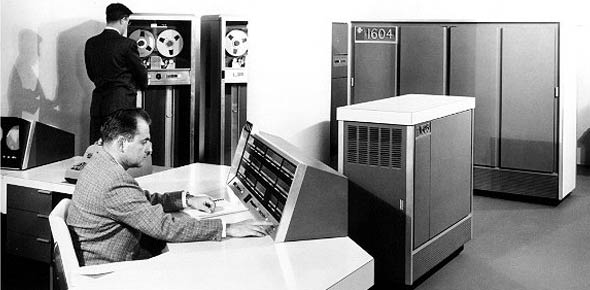# Vol2 Ure2 CDC 3D153

26 Questions | Total Attempts: 114SettingsThis is a URE #2 for CDC 3D153 and is to be used as a study aid for the RF transmission systems CDC's.

• 1.
(213) Direct waves are radio waves that travel
• A.

Long distances with few interruptions

• B.

From point to point along the earth’s surface

• C.

Through the air in a straight line.

• D.

No more than 20 miles to the receive antenna

• 2.
(213) Radio waves that travel near the earth’s surface are called
• A.

Earth waves

• B.

Global waves

• C.

Ground waves

• D.

Terrestrial waves

• 3.
(213) Sky wave transmission is used for which type of communications?
• A.

Long distance

• B.

Subterrestrial

• C.

Atmospheric

• D.

Line of sight

• 4.
(214) Which gives sky wave propagation its ability to communicate beyond the optical line-of sight (LOS)?
• A.

Refraction

• B.

Reflection

• C.

Earth's conductivity

• D.

Atmospheric charge

• 5.
(214) Which gives sky wave propagation its ability to communicate beyond the optical line-ofsight (LOS)?
• A.

Refraction

• B.

Reflection

• C.

Earth's conductivity

• D.

Atmospheric change

• 6.
(214) Frequencies higher than the critical frequency are?
• A.

Returned to earth

• B.

Passed into space

• C.

The most desirable

• D.

Refracted by the F2 layer

• 7.
(214) In high frequency (HF) communications, the lowest frequency that arrives at the distant receiver on 90 percent of the undisturbed days of the month is called the?
• A.

Maximum usable frequency (MUF)

• B.

Lowest usable frequency (LUF)

• C.

Operating frequency

• D.

Critical frequency

• 8.
(214) The abbreviation FOT is derived from the term?
• A.

Outbound traffic frequency

• B.

Optimum tropospheric frequency

• C.

Frequency of optimum transmission

• D.

Frequency of outbound frequency

• 9.
(214) Magneton splitting creates two waves called?
• A.

Direct and indirect

• B.

Ordinary and abnormal

• C.

In-phase and out-of-phase

• D.

Ordinary and extraordinary

• 10.
(214) What occurs when a transmitted signal travels over two or more separate paths during transmission?
• A.

Skip effects

• B.

Modulation

• C.

Multipathing

• D.

Magneton splitting

• 11.
(215) Which layer of the ionosphere is most important to high frequency (HF) communications?
• A.

D

• B.

E

• C.

F

• D.

Topside

• 12.
(215) During what season do we have the wider range of critical frequencies and less absorption of all frequencies?
• A.

Fall

• B.

Winter

• C.

Spring

• D.

Summer

• 13.
(215) What is the recombination process dependent upon?
• A.

Season

• B.

Time of day

• C.

Regular variation

• D.

Irregular variations

• 14.
(215) Sunspots are disturbances?
• A.

In the sun's atmosphere

• B.

On the sun's surface

• C.

In the ionosphere

• D.

Beneath the sun's surface

• 15.
(216) For communications purposes, the usable frequency spectrum ranges from?
• A.

3Hz to 300 GHz+

• B.

300 Hz to 3000 GHz

• C.

3000 Hz to 3000 GHz

• D.

30 MHz to 300 MHz

• 16.
(216) What U.S. government agency divides the radio frequency (RF) spectrum into different bands?
• A.

Federal Aviation Agency

• B.

American National Standard Institute

• C.

Federal Communications Commission

• D.

Institute of Electrical & Electronics Engineers, INC

• 17.
(217) Near-vertical-incidence sky (NVIS) wave operations are most effective when you’re using?
• A.

Lower high frequency (HF) frequencies

• B.

Higher HF frequencies

• C.

Medium HF frequencies

• D.

Low takeoff antenna angles

• 18.
(217) What frequency band is most susceptible to jamming?
• A.

Low frequency

• B.

Medium frequency

• C.

High Frequency

• D.

Very High Frequency

• 19.
(217) What frequency bands doe the MILSTAR satellite system use?
• A.

High frequency (HF) and Ultra high Frequency (UHF)

• B.

Super-High Frequency (SHF) and Extremely high frequency (EHF)

• C.

Very high frequency (VHF) and SHF

• D.

VHF and UHF

• 20.
(218) The main very high frequency (VHF) Ultra high frequency (UHF) communications emphasis is on?
• A.

Long range deployable communications

• B.

Short range fixed communications

• C.

Emergency communications

• D.

Air-ground communications

• 21.
(219) Super high frequency (SHF)/extremely high frequency (EHF) range is limited to?
• A.

Scattering

• B.

Line-of-sight (LOS)

• C.

Earth's conductivity

• D.

Atmospheric absorption

• 22.
(220) In a move to overcome the limited range of forward propagation by tropospheric scatter (FPTS) without requiring many costly relay systems, what was developed?
• A.

• B.

Satellite communications

• C.

Microwave communications

• D.

Line-of-sight (LOS) communication

• 23.
(220) Why isn’t very high frequency (VHF) normally used for satellite communications?
• A.

The frequency is too low

• B.

The frequency is too high

• C.

Ionospheric refraction can occur

• D.

The rate of absorption is too great

• 24.
(220) When comparing the radio and optical horizons, which one is farther away and by what percent?
• A.

Optical; 15

• B.

• C.

Optical; 33

• D.

• 25.
(220) When K is greater than 4/3, the radio horizon will?
• A.

Decrease, then increase.

• B.

Remain the same

• C.

Decrease

• D.

Increase

Related TopicsBack to top